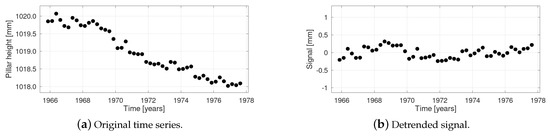Next Article in Journal
An Efficient Method for Forming Parabolic Curves and Surfaces
Next Article in Special Issue
Elementary Error Model Applied to Terrestrial Laser Scanning Measurements: Study Case Arch Dam Kops
Previous Article in Journal
Theoretical Aspects on Measures of Directed Information with Simulations
Previous Article in Special Issue
Variance Reduction of Sequential Monte Carlo Approach for GNSS Phase Bias Estimation
Open AccessArticle

# A Generic Approach to Covariance Function Estimation Using ARMA-Models

byTill Schubert *,Johannes Korte,Jan Martin BrockmannandWolf-Dieter SchuhInstitute of Geodesy and Geoinformation, University of Bonn, 53115 Bonn, Germany
*
Author to whom correspondence should be addressed.
Mathematics 2020, 8(4), 591; https://doi.org/10.3390/math8040591
Received: 27 February 2020 / Revised: 9 April 2020 / Accepted: 11 April 2020 / Published: 15 April 2020
(This article belongs to the Special Issue Stochastic Models for Geodesy and Geoinformation Science)
Covariance function modeling is an essential part of stochastic methodology. Many processes in geodetic applications have rather complex, often oscillating covariance functions, where it is difficult to find corresponding analytical functions for modeling. This paper aims to give the methodological foundations for an advanced covariance modeling and elaborates a set of generic base functions which can be used for flexible covariance modeling. In particular, we provide a straightforward procedure and guidelines for a generic approach to the fitting of oscillating covariance functions to an empirical sequence of covariances. The underlying methodology is developed based on the well known properties of autoregressive processes in time series. The surprising simplicity of the proposed covariance model is that it corresponds to a finite sum of covariance functions of second-order Gauss–Markov (SOGM) processes. Furthermore, the great benefit is that the method is automated to a great extent and directly results in the appropriate model. A manual decision for a set of components is not required. Notably, the numerical method can be easily extended to ARMA-processes, which results in the same linear system of equations. Although the underlying mathematical methodology is extensively complex, the results can be obtained from a simple and straightforward numerical method. View Full-Text
Show FiguresFigure 1

MDPI and ACS Style

Schubert, T.; Korte, J.; Brockmann, J.M.; Schuh, W.-D. A Generic Approach to Covariance Function Estimation Using ARMA-Models. Mathematics 2020, 8, 591.